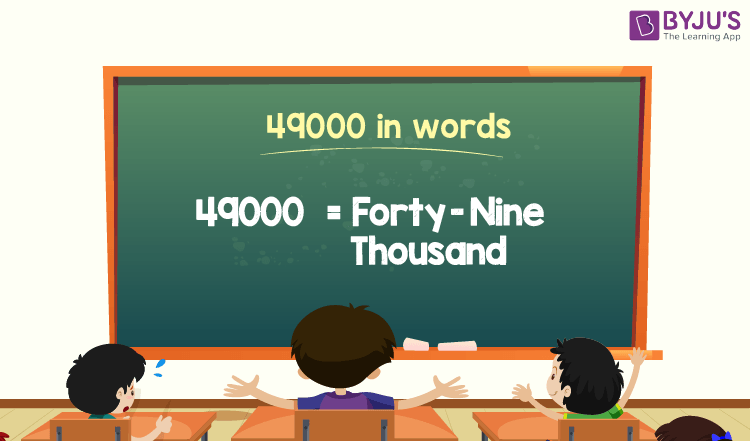# 49000 in Words

49000 in words is Forty-nine Thousand. For example, if you earned 49000 this month, you can write, “I have earned forty-nine thousand this month”. The place value chart helps to write the number name of 49000.

 49000 in Words: Forty-nine Thousand

In this article, we will discuss how to write the number 49000 in words, and look at the solved examples in detail.

## How to Write 49000 in Words?The number 49000 in words is written using the place value of a number. Thus, to write 49000 in words, find the place value of each digit of 49000.

For a number 49000,

In one’s place, the digit is 0.

In ten’s place, the digit is 0.

In hundred’s place, the digit is 0.

In thousand’s place, the digit is 49.

Thus, the place value chart that represents 49000 is as follows:

 Thousands Hundreds Tens Ones 49 0 0 0

Therefore, the number 49000 in words is Forty-nine Thousand.

### Examples

Example 1:

Express the value of 50000 – 1000 in words.

Solution:

Given expression: 50000 – 1000

⇒ 50000 – 1000 = 49000

So, the value of 50000 – 1000 is 49000.

Hence, 49000 in words is forty-nine thousand.

Example 2:

Find the value of forty-nine thousand plus ten thousand and express it in words.

Solution:

Forty-nine thousand = 49000

Ten thousand = 10000

Forty-nine thousand plus ten thousand = 49000 + 10000= 59000

Hence, the value of forty-nine thousand plus ten thousand is fifty-nine thousand in words.

## Frequently Asked Questions on 49000 in Words

Q1

### Write 49000 in words.

49000 in words is forty-nine thousand.

Q2

### Find the value of 49500 – 500, and express the answer in words.

Simplifying 49500 – 500, we get 49000. Hence, 49000 in words is forty-nine thousand.

Q3

### How to write forty-nine thousand in numbers?

Forty-nine thousand in numbers is 49000.